### 3D Parameter Window – OpenGL – Object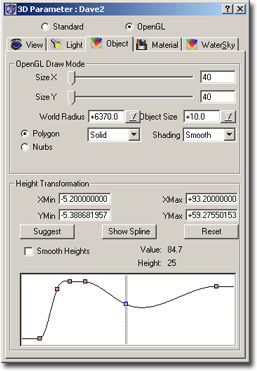Fig.: 3D Parameters – Object Tab

This tab lets you define how ChaosPro creates the 3D fractal object from the color values.

First of all, remember what ChaosPro already has calculated and what needs to be done:
ChaosPro already has:

• A buffer containing color values exactly the size you specified in the size window.

Now ChaosPro needs to create heights from this buffer with its color values. ChaosPro could calculate a height for each value in the buffer, resulting in a second buffer of the same size with all heights. Ok, but this would be too slow for OpenGL because the system then has to shade so many polygons that you need a quite fast computer to get an acceptable speed.

So you first need to specify the size of the height buffer: You do this by using the two sliders SizeX and SizeY, which let you adjust the size of the height buffer upto the size of the original color value buffer. In the image above the size is set to 40×40, whereas the color value buffer has the size 320×256: That means, in x-direction only every 8th color value is taken, in y-direction every 6th color value is taken.

After that you can specify the world radius and the size of the fractal object: The object is placed on a sphere having the specified radius. Normally the sphere radius is 6370 (i.e. the radius in kilometers of the earth). The fractal object size is 10, which means, it has radius 10, which means its width is about 20 units (kilometers), 10 units to the left and 10 units to the right.

By choosing the radius of the world small and the object size similar to the world radius it is possible to wrap the fractal object around the sphere.

OpenGL Draw Mode

After the world radius and object size there are settings which specify the OpenGL draw mode:

• Polygon: In this mode polygons are created using the coordinates of the height buffer. The following draw modes exist: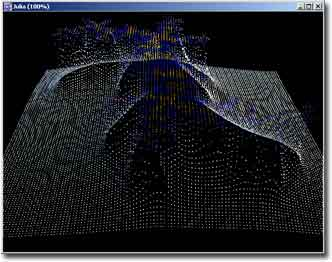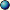Point : Only points are drawn (i.e. the corners of the polygons), you can specify the point size.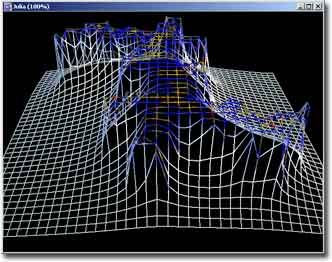Line : Only lines are drawn (i.e. the lines of all polygons, but the polygons are not filled), you can specify the line width. This mode is also called wire-frame.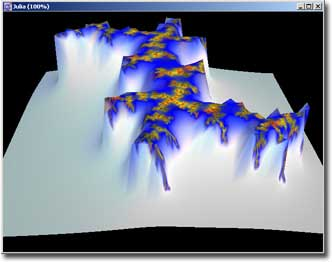Solid : Whole filled polygons are drawn.
• Nurbs: This mode takes the coordinates of the height buffer and calculates many small NURBs (non uniform rational b-spline). A nurb surface is a smooth interpolation surface. In order to actually draw the nurb it has to be triangulated, i.e. many small triangles have to be calculated which approximate the NURB. OpenGL does this automatically, but it expects an additional parameter called tolerance, which specifies a maximum error: If a triangle generates an approximation error larger than this value, it gets divided into smaller triangles until the approximation error is smaller than the maximum error.
You can choose between the following draw modes:Point : Just draws the corner points of each NURB.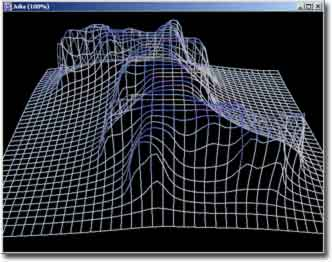Patch : Draws the outline of each NURB (similar to wire frame, but interpolated).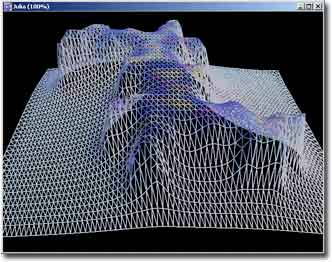Polygon : Draws the wire frame model of each triangulated NURB: Here you can see all trinagles which OpenGL automatically generates in order to approximate the NURB using the tolerance: Adjust the tolerance and you’ll see that a smaller tolerance produces more triangles (slowing down the calculation), a larger tolerance leads to fewer triangles (speeding up the calculation).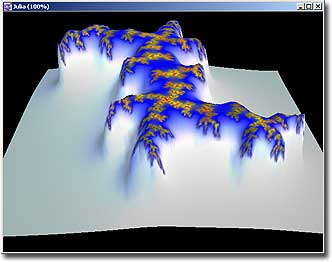Solid : Draws the filled NURB.

For each mode above you can choose the shade type: Either “Flat” (a single polygon has a single color) or “Smooth” (OpenGL interpolates the colors in the polygon).

Height Transformation

The lower half of the window contains the definition of the height transformation spline: It lets you view and change the spline function responsible for calculating the height from a color value, it lets you define how buffer values are transformed into heights.
The easiest method is simply that height of pixel = color number. But this transformation is useless due to the nature of fractals. So a spline is used in order to increase flexibility.

The graph displays knots, and you can move these knots using either the mouse or the keyboard (cursor keys, page up/down). You can add a knot by double clicking to the desired location, you can delete knots by selecting them and pressing the “Delete” key on your keyboard.

The different knots then are interpolated using a cubic spline. This method lets you easily change the transformation function.
This method is very powerful. If you have increasing buffer / color values, you may decrease the heights assigned to those values, which may result in a nice valley appearing instead of a plateau.

The other fields above the display field are:

• XMin, XMax, YMin, YMax
These values simply define the scale of the graph display area below.
• Suggest
Well, if you played around with these values, then you may have noticed, that it is quite difficult to get a good starting point. Often the 3D view only consists of spikes. Obtaining a good starting point is the biggest challenge. I teached ChaosPro to calculate a suggestion. So if you click onto the button Suggest, then ChaosPro will try to find a good starting point.
• Show Spline
This button changes the scale so that the whole spline and all knots are visible.
• Reset
Resets the scale to XMin=0, XMax=200, YMin=0, YMax=100.
• Smooth heights
If selected, the color value buffer gets smoothe
d: This reduces spikes.